Test: Automatic Control Systems - 6

# Test: Automatic Control Systems - 6

Test Description

## 25 Questions MCQ Test | Test: Automatic Control Systems - 6

Test: Automatic Control Systems - 6 for Electrical Engineering (EE) 2023 is part of Electrical Engineering (EE) preparation. The Test: Automatic Control Systems - 6 questions and answers have been prepared according to the Electrical Engineering (EE) exam syllabus.The Test: Automatic Control Systems - 6 MCQs are made for Electrical Engineering (EE) 2023 Exam. Find important definitions, questions, notes, meanings, examples, exercises, MCQs and online tests for Test: Automatic Control Systems - 6 below.
Solutions of Test: Automatic Control Systems - 6 questions in English are available as part of our course for Electrical Engineering (EE) & Test: Automatic Control Systems - 6 solutions in Hindi for Electrical Engineering (EE) course. Download more important topics, notes, lectures and mock test series for Electrical Engineering (EE) Exam by signing up for free. Attempt Test: Automatic Control Systems - 6 | 25 questions in 25 minutes | Mock test for Electrical Engineering (EE) preparation | Free important questions MCQ to study for Electrical Engineering (EE) Exam | Download free PDF with solutions
 1 Crore+ students have signed up on EduRev. Have you?
Test: Automatic Control Systems - 6 - Question 1

### A phase lead network with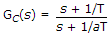where a < 1, gives maximum phase lead at a frequency equal to

Test: Automatic Control Systems - 6 - Question 2

### Generally a servomotor operates at

Test: Automatic Control Systems - 6 - Question 3

### If C is thermal capacitance, m is mass of liquid and c is specific heat, then

Test: Automatic Control Systems - 6 - Question 4

Assertion (A): The concept of impulse function is useful in differentiating discontinuous functions.

Reason (R):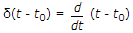Test: Automatic Control Systems - 6 - Question 5

In a two phase servomotor, if R is rotor resistance, X is rotor reactance, L is rotor length D is rotor diameter then

Test: Automatic Control Systems - 6 - Question 6

The ac motor used in servo applications is

Test: Automatic Control Systems - 6 - Question 7

For the log magnitude Bode plot of the given figure, the transfer function is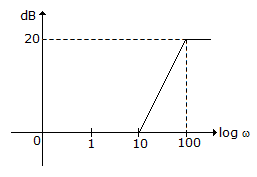Test: Automatic Control Systems - 6 - Question 8

A first-order system has a time constant t. For unit ramp input, the steady state error is

Test: Automatic Control Systems - 6 - Question 9

The system in the given figure is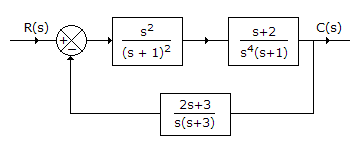Test: Automatic Control Systems - 6 - Question 10

For the equation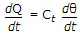where Q is heat energy, Ct is thermal capacitance and θ is temperature, the analogous electrical equation is

Test: Automatic Control Systems - 6 - Question 11

Optical encoders most commonly used in control systems are

Test: Automatic Control Systems - 6 - Question 12

A second order system exhibits 100% overshoot. The damping ratio is

Test: Automatic Control Systems - 6 - Question 13

The log magnitude curve for a constant gain K is a horizontal straight line at a magnitude 20 log K dB.

Test: Automatic Control Systems - 6 - Question 14

For the plot in the given figure, G(jω) =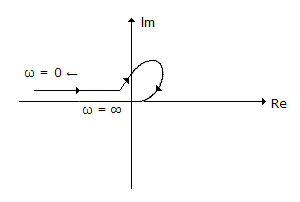Test: Automatic Control Systems - 6 - Question 15

Transfer functions of even complicated components can be found by frequency response tests.

Test: Automatic Control Systems - 6 - Question 16

The shape of torque-speed curve of a two phase servo- motor mostly depends on

Test: Automatic Control Systems - 6 - Question 17

In a lag network the input frequency is 100 fc where fc is critical frequency. The voltage gain is

Test: Automatic Control Systems - 6 - Question 18

Assertion (A): Temperature control of the passenger compartment of car uses both feed forward and feedback controls.

Reason (R): Feed forward control gives corrective action before the disturbance affects the output and feedback control applies corrective action after output changes.

Test: Automatic Control Systems - 6 - Question 19

The correct sequence of events to improve system stability is

Test: Automatic Control Systems - 6 - Question 20

Assertion (A): Most of actual systems are non-linear.

Reason (R): Non linearity may be due to saturation, dead zone, square law etc.

Test: Automatic Control Systems - 6 - Question 21

The static equation of a system is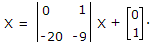The poles of this system are located at

Test: Automatic Control Systems - 6 - Question 22

The pole-zero configuration of the given figure can be for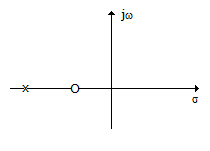Test: Automatic Control Systems - 6 - Question 23

Which of the following represents constant magnitude locus for M = 1?

Test: Automatic Control Systems - 6 - Question 24

Assertion (A): A synchro control transformer has nearly constant impedance across its rotor terminals.

Reason (R): Rotor of a synchro control transformer is cylindrical so as to give uniform flux distribution.

Test: Automatic Control Systems - 6 - Question 25

The log magnitude plot of the given figure is for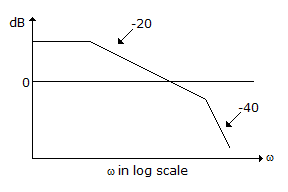Use Code STAYHOME200 and get INR 200 additional OFF Use Coupon Code
Information about Test: Automatic Control Systems - 6 Page
In this test you can find the Exam questions for Test: Automatic Control Systems - 6 solved & explained in the simplest way possible. Besides giving Questions and answers for Test: Automatic Control Systems - 6, EduRev gives you an ample number of Online tests for practice